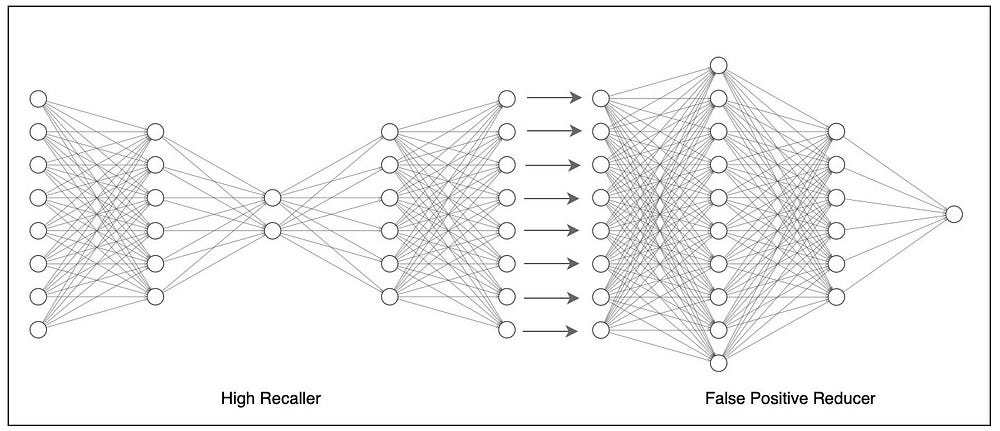# Autoencoder Neural Network for Anomaly Detection with Unlabeled Dataset

Source: Deep Learning on Medium# Autoencoder Neural Network for Anomaly Detection with Unlabeled Dataset

If you are trying to detect anomalies from an unlabeled dataset and you are worried about not having a labeled dataset, then here is an interesting solution. All you need is an imbalanced dataset with nearly 1% of the anomalies.

# Autoencoder Neural Network

In contrast to a normal neural network, autoencoder neural network has the same number of neurons in the output layer as the input layer. The output which it tries to predict is also the same as the input. Yes, the job of an autoencoder neural network is encoding the data into a small code and decoding it back to reproduce the input. This particular property enables us to use it for an unlabeled dataset. Autoencoder neural networks are commonly used for dimensionality reduction in computer vision to natural language processing. To understand more about autoencoder neural networks, have a read on this Wikipedia page.

## How this is possible for an unlabeled dataset?

Since we are trying to reproduce the input with this model, the loss function that suits the best is mean square error (MSE). While training the model, what it tries to do is to minimize the MSE. To minimize MSE it should try to fit the dataset much as possible (i.e) it should try to reproduce many data as possible. In our case, since the dataset consists 99% of normal data and only 1% of anomalies, what happens while training is, the model misses out the small proportion and fits the remaining 99% of the data so that the MSE is very very small. This is the core logic behind, which makes it possible to use it to predict the anomalies even for unlabeled datasets.

## How to predict the anomalies?

While training the model it learns how the features will look like for normal data and compress it into a small element and decode it back as the input with a small error. When an anomaly is sent through the model, it will fail to reproduce it, since it is trained to reproduce only normal data and will end up with a large MSE. What we need to do is calculate MSE of the output compared to input and to properly differentiate the anomalies, by checking the outputs we need to set a threshold value for MSE according to our need so that it predicts with good precision and recall.

# That’s not All

Most of the time it is difficult to achieve fully satisfying results by keep tuning the hyper-parameters and checking the outputs to come up with the best threshold value. But we have more options.

## The High Recaller

Even if we are training it with thousands of data, what we will get as anomalies is few hundreds. So now humanly it is possible to manually go through the anomaly outputs and flag some of them as false positives. Therefore, we can let our autoencoder neural network model as a High Recaller. That means keeping the threshold low so that almost all the actual anomalies are getting detected (high recall) along with other false positive anomalies (low precision).

Now what we have is a small dataset that can be manually flagged as false and keep the rest as true. For this manually labeled dataset, let’s introduce a new artificial neural network model.

## The False Positive Reducer

With the dataset now we have we can choose a neural network or based on the complexity, even a random forest or any other typical ML model and we can simply train it. This enables precise prediction of actual anomalies we want to be predicted by eliminating the false positives in the final output.

## Prediction in Real-time

All we need to do now is stacking up these models so that in real-time prediction those which are predicted as anomalies by the high recaller model (autoencoder neural network) are sent through the false positive reduction model (artificial neural network). This combination of neural networks together gives us a deep neural network model with high recall and high precision.